Function Repository Resource:

# SwapOrderedPermutations

Construct a list of permutations so that successive permutations differ by exactly one transposition

Contributed by: Wolfram Staff (original content by Sriram V. Pemmaraju and Steven S. Skiena)
 ResourceFunction["SwapOrderedPermutations"][l] constructs all permutations of list l such that adjacent permutations differ by only one transposition.

## Details and Options

A transposition is a swap of two elements of an ordered list with all others staying at the their respective positions.
ResourceFunction["SwapOrderedPermutations"] uses Heap’s algorithm.

## Examples

### Basic Examples (2)

Compute a full list of permutations each differing from the previous permutation by exactly one transposition:

 In:=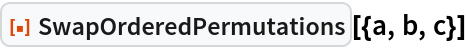Out=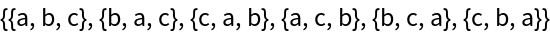Find swap-ordered permutations for four elements:

 In:=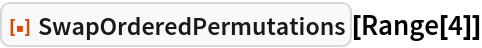Out=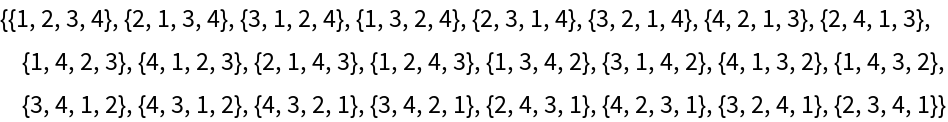### Properties and Relations (2)

SwapOrderedPermutations differs from Permutations in the ordering of the result:

 In:=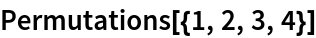Out=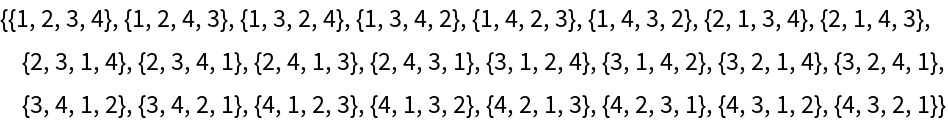In:=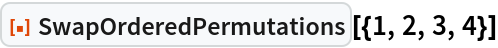Out=In:=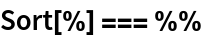Out=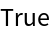SwapOrderedPermutations defines a Hamiltonian path in the transposition graph:

 In:=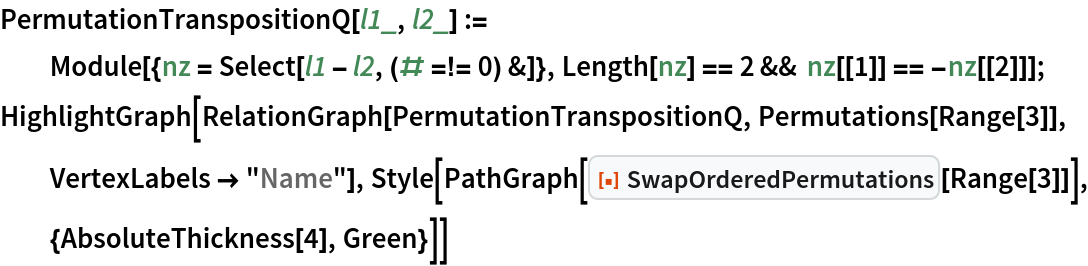Out=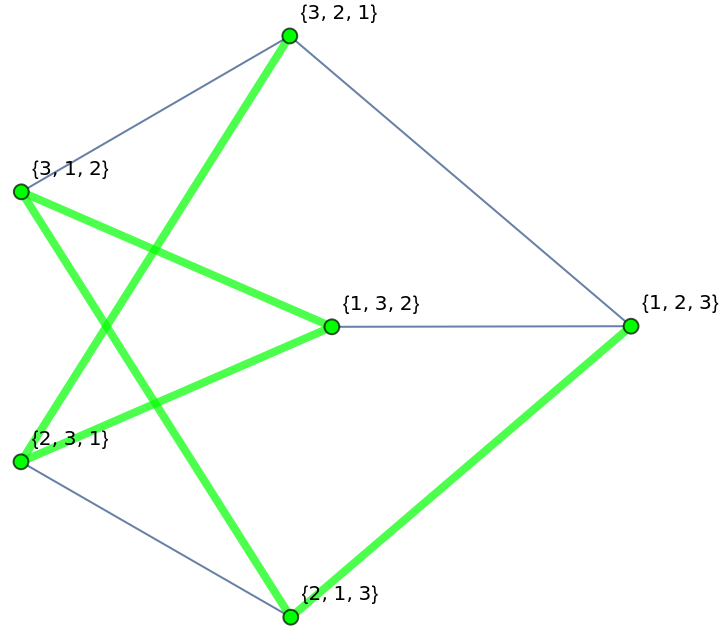## Version History

• 1.0.0 – 27 August 2020